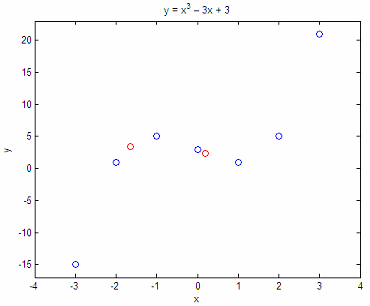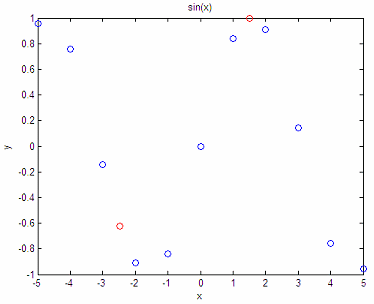# (curvilinear interpolation)

The computations in this small article show the Lagrange interpolation. The code computes y-coordinates of points on a curve given their x-coordinates.

You must enter coordinates of known points on the curve, no two having the same abscissa.

This is the simple function:

function y0 = lagrange_interp(x, y, x0)
% x is the vector of abscissas.
% y is the matching vector of ordinates.
% x0 represents the target to be interpolated
% y0 represents the solution from the Lagrange interpolation

y0 = 0;
n = length(x);

for j = 1 : n
t = 1;

for i = 1 : n

if i~=j
t = t * (x0-x(i))/(x(j)-x(i));

end
end
y0 = y0 + t*y(j);
end

### Example 1 - Interpolate a cubic function

Consider the curve y = x3 - 3x + 3. We now that points

x = [-3 -2 -1 0 1 2 3];
y = [-15 1 5 3 1 5 21];

are on the curve. What are the values of y when x = -1.65 and 0.2?

x1 = -1.65;
y1 = lagrange_interp(x,y,x1)

x2 = .2;
y2 = lagrange_interp(x,y,x2)

The results are:
y1 = 3.4579
y2 = 2.4080

Let’s plot our approach:

plot(x, y, 'bo', x1, y1, 'ro', x2, y2, 'ro')
axis([-4 4 -17 23])

title(‘y = x^3 – 3x + 3’)
xlabel(‘x’)
ylabel(‘y’)### Example 2 -  Interpolate a sine function

Given the following points from a sine curve, what are the y-values for x = -2,47 and x = 1.5?

x = [-5 -4 -3 -2 -1 0 1 2 3 4 5];
y = [.958 .757 -.141 -.909 -.841 0 .841 .909 .141 -.757 -.959];

x3 = -2.47;
y3 = lagrange_interp(x,y,x3)

x4 = 1.5;
y4 = lagrange_interp(x,y,x4)

The results are:

y3 = -0.6218
y4 =  0.9972

And our plot is:

plot (x, y, 'bo', x3, y3, 'ro', x4, y4, 'ro')
title(
'sin(x)')
xlabel(
'x')
ylabel(
'y')The approximation is not bad, right? In fact, it seems to be quite accurate!

From Lagrange Interpolation to home

From Lagrange Interpolation to Generic Programming